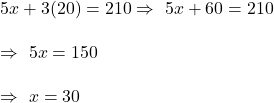## The cost for 5 adults and 3 children to attend a show is $210. ( /5) For 2 adults and 5 children the cost is$160. Form a pair o

Question

The cost for 5 adults and 3 children to attend a show is $210. ( /5) For 2 adults and 5 children the cost is$160.
Form a pair of simultaneous linear equations and solve them to find the cost of:
ii. a child ticket

in progress 0
4 months 2021-08-18T07:01:07+00:00 1 Answers 0 views 0

1. Answer: i. Cost of adult ticket = $30 ii. Cost of child ticket =$20

Step-by-step explanation:

Define variables:

Let x= cost of 1 adult tickets , y= cost of 1 child tickets.

Form linear equations:

5x+3y = 210      (i)

2x+5y= 160  (ii)

Multiply (i) by 2 and (ii) by 5

10x +6y = 420       (iii)

10x+25y = 800        (iv)

Eliminate (iii) from (iv)

19y = 380

⇒ y= 20

Put this in (i)Hence, Cost of adult ticket = $30 and Cost of child ticket =$20